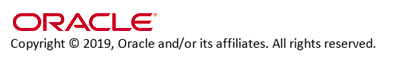Costing Method (A25)

Purpose: Use this screen to define how the cost of an item is calculated.

Code field: Enter the code for the costing method you will use for inventory.

The inventory costing methods are:

• AVERAGE

• STANDARD

• FIFO

The costing method you select determines how you determine the cost of each item.

Average costing method: If you use the AVERAGE inventory costing method, the system calculates a new average cost automatically during Purchase Order Receiving, and updates the Cost field in the SKU table with the new, calculated average cost.

Average cost calculation:

(current on-hand x current average cost) + (quantity received x new purchase cost) / (quantity on-hand + quantity received) = new average cost

The new purchase cost represents the actual cost at which the item was purchased plus any overhead charges and additional item charges. See Purchase Order Receiving Overview for more information.

For example:

current on-hand = 185

current average cost = 25.00

new purchase cost = 28.75 (25.00 + 15% for commission)

(185 x 25.00) + 25 x 28.75) / (185 + 25) = 25.45 (new average cost)

The system continues to change the cost of the item as the system factors the cost of the item at Receiving time. This field is updated only during Purchase Order Receipts.

Standard costing method: If you use the STANDARD inventory costing method, the Cost field in the SKU table must be updated manually; the system does not calculate or assign a cost to this field automatically.

Storing costs: The system always calculates and stores the average cost of an item for future reference, regardless of the default costing method you choose. Once you establish this control value, you can change it to the other costing method in order to generate special reports or perform special analyses.

FIFO costing: FIFO (first-in, first-out) costing allows you to use track the different costs assigned to each quantity of an item at the time you receive it into your warehouse. For example, if you receive 10 units of an item at \$1.00, then 5 units of the item at \$1.50, the system uses a cost of \$1.00 for the first 5 units you sell, then uses a cost of \$1.50 for the next 5.

Important: Unless you are first setting up a new company (that is, there has not been any inventory activity at all), you cannot change to or from FIFO costing at the Costing Method screen; instead, you must run a conversion program to convert to or from the different table requirements of FIFO costing. See FIFO Costing Overview for more information on this conversion program and for an overview of how FIFO costing works.IN03_03 OMSCS 19.0 December 2019 OHC